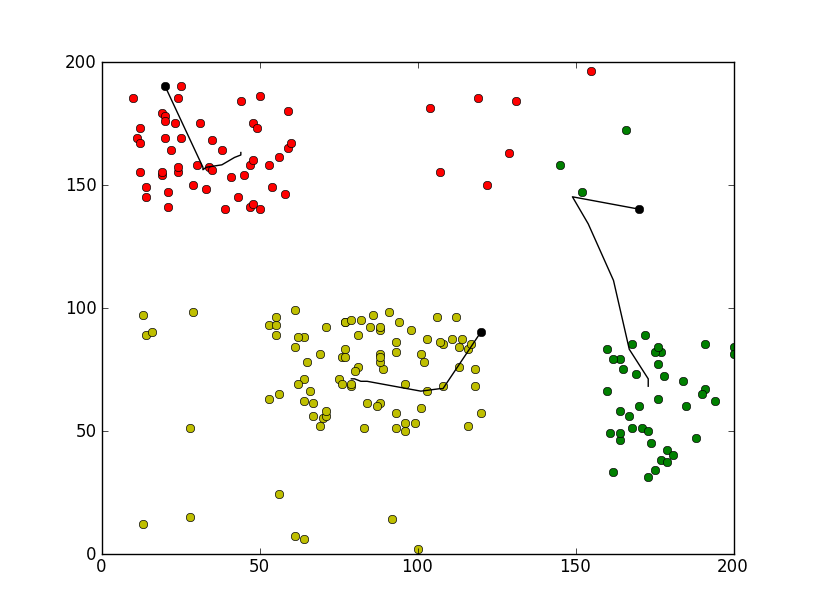# 初识聚类算法: 基本K均值

intergret 发布于 2012年10月22日 21时, 0评/3153阅

基本K均值 ：选择 K 个初始质心，其中 K 是用户指定的参数，即所期望的簇的个数。每次循环中，每个点被指派到最近的质心，指派到同一个质心的点集构成一个。然后，根据指派到簇的点，更新每个簇的质心。重复指派和更新操作，直到质心不发生明显的变化。
详见博客：http://blog.sina.com.cn/s/blog_62186b460101ard2.html

## 代码片段(2)[全屏查看所有代码]

### 1. [代码][Python]代码     跳至  [全屏预览]

```# scoding=utf-8
import pylab as pl

points = [[int(eachpoint.split("#")), int(eachpoint.split("#"))] for eachpoint in open("points","r")]

# 指定三个初始质心
currentCenter1 = [20,190]; currentCenter2 = [120,90]; currentCenter3 = [170,140]

pl.plot([currentCenter1], [currentCenter1],'ok')
pl.plot([currentCenter2], [currentCenter2],'ok')
pl.plot([currentCenter3], [currentCenter3],'ok')

# 记录每次迭代后每个簇的质心的更新轨迹
center1 = [currentCenter1]; center2 = [currentCenter2]; center3 = [currentCenter3]

# 三个簇
group1 = []; group2 = []; group3 = []

for runtime in range(50):
group1 = []; group2 = []; group3 = []
for eachpoint in points:
# 计算每个点到三个质心的距离
distance1 = pow(abs(eachpoint-currentCenter1),2) + pow(abs(eachpoint-currentCenter1),2)
distance2 = pow(abs(eachpoint-currentCenter2),2) + pow(abs(eachpoint-currentCenter2),2)
distance3 = pow(abs(eachpoint-currentCenter3),2) + pow(abs(eachpoint-currentCenter3),2)

# 将该点指派到离它最近的质心所在的簇
mindis = min(distance1,distance2,distance3)
if(mindis == distance1):
group1.append(eachpoint)
elif(mindis == distance2):
group2.append(eachpoint)
else:
group3.append(eachpoint)

# 指派完所有的点后，更新每个簇的质心
currentCenter1 = [sum([eachpoint for eachpoint in group1])/len(group1),sum([eachpoint for eachpoint in group1])/len(group1)]
currentCenter2 = [sum([eachpoint for eachpoint in group2])/len(group2),sum([eachpoint for eachpoint in group2])/len(group2)]
currentCenter3 = [sum([eachpoint for eachpoint in group3])/len(group3),sum([eachpoint for eachpoint in group3])/len(group3)]

# 记录该次对质心的更新
center1.append(currentCenter1)
center2.append(currentCenter2)
center3.append(currentCenter3)

# 打印所有的点，用颜色标识该点所属的簇
pl.plot([eachpoint for eachpoint in group1], [eachpoint for eachpoint in group1], 'or')
pl.plot([eachpoint for eachpoint in group2], [eachpoint for eachpoint in group2], 'oy')
pl.plot([eachpoint for eachpoint in group3], [eachpoint for eachpoint in group3], 'og')

# 打印每个簇的质心的更新轨迹
for center in [center1,center2,center3]:
pl.plot([eachcenter for eachcenter in center], [eachcenter for eachcenter in center],'k')

pl.show()```

### 2. [图片] Kmeans.pngintergret的其它代码